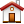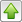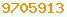# binary : Java Glossary

binary
Base two numbers, made up only of the digits zero and one. Internally this in what computer chips use to calculate, where a high voltage represents 1 and a low voltage 0. With one wire, you have only two possibilities low and high, 0 and 1. With two wires you can represent 4 possibilities, 00, 01, 10, 11. With three wires you can represent 8 possibilities, 000, 001, 010, 011, 100, 101, 110, 111. You can assign these possibilities to the integers: 0=000, 1=001, 2=010, 3=011, 4=100, 5=101, 6=110, 7=111. The scheme works much like decimal arithmetic. The column values are :… 64 32 16 8 4 2 1 (i.e. 2^n). Thus 1011 is 1*8 + 0*4 + 2*1 + 1*1 = 11 — decimal eleven.

## Signed Binary

Since computers only have a limited number of bits, they use modulo arithemetic, where the modulus is 2^n and n is the bitsize. All results have to fit in the range 0 .. 2^n - 1. As a consequence, negative numbers are usually stored as if they were large positive numbers. In Java, negative numbers use 2’s-complement notation. (Other languages also support older mainframe computers that use 1’s-complement.)

For one’s complement, (Java unary ~ operator) take the bit pattern for the equivalent positive number, invert all bits 10 and 01, e.g. ~5 is 0000010111111010.

For two’s complement, (Java unary - operator) take the bit pattern for the equivalent positive number, invert all bits 10 and 01, then add one, e.g. -5 is 000001011111101011111011.

Binary is rather bulky to write out, so instead it is often written in terser hexadecimal (hex) or sometimes octal. Unfortunately, there is no way in Java prior to 1.7 to include binary literals in programs other than by encoding them in hex or the more old fashioned octal. In version 1.7+ you can write them with a lead 0b, the same way you can write hex with a lead 0x. Further, you can use _ to group digits e.g. 0b1010_1100.

## Magic Numbers

When you realise that 255 = 1111_1111 in binary, 1024 = 100_0000_0000 and 65535 = 1111_1111_1111_1111, you might guess why these numbers tend to come up so often in computing.

## Bit Manipulating

Often bit manipulations are attempting to pack several fields into a single int and then unpack them again. You do this work with >>>, <<, &, | and ~. Rarely you might use the signed shift operator >>. For example to extract the low order three bits you mask with binary 00000111, e.g. z = x & 0x07. To extract bits 4 and 5 you shift and mask, e.g. z = x >>> 4 & 0x03. To put together a 2-bit x field in bits 4 and 5 and a 3-bit y field in bits 0, 1, 2 you use code like this: z = x << 4 | y. To zero out a the x field you would take the mask for bits 4 and 5, binary 110000 and invert it and then mask with that, e.g. z &= ~0x30;

Common boolean operators include & (bitwise AND for masking), | (bitwise OR for combining), >> (right signed shift), >>> (right unsigned shift), << (left signed/unsigned shift), ~ logical not and ^ bitwise xor. Unfortunately Java does not support binary literals 0b111 or underscores as shown in the examples. You must use hexadecimal notation without underscores instead.

## Bitwise Operators

Bitwise Operators
binary operation result notes
0b0101_0101 & 0b0001_1100 0b0001_0100 & logical AND, logical carryless bitwise multiply, used for masking (getting rid of parts of a word you don’t want), 1s where both operands have a 1 otherwise 0. Don’t confuse this with &&.

0 & 00
0 & 10
1 & 00
1 & 11

## & Tips

• Given that you have a mask to describe the bit (all zeros, one one), to turn off a bit, use & ~mask .
• A quick way to determine if n is odd is to use the expression (n&1) != 0.
• (n & -n) is the highest power of two that is a divisor of n. It finds the rightmost one bit and isolates it.
• A quick way to calculate the remainder modulo 16 is (n&15). The n &(m-1) trick works for any power of 2. If any negative numbers are involved you will get different answers from Java’s %, but possibly still useful ones.
• You can use & to quickly determine if a number is a power of two:
`boolean isPowerOfTwo = (n & -n) == n;`
• To do the inverse, compute how many bits wide a number is,
• Normally you create masks with hex literals, e.g. 0x007f. To dynamically create a mask with n low order 1’s, use (1<<n)-1. Beware, for int this will not work for n=32 since shifts are done modulo 32.
0b1110_0000 | 0b1000_0001 0b1110_0001 | logical OR, logical carryless bitwise addition, 1s where either operand has a 1, otherwise 0. Useful for combining bit masks. Don’t confuse this with ||. Given that you have a mask to describe the bit (all zeros, one one), to turn on a bit, use | mask.

0 | 00
0 | 11
1 | 01
1 | 11

0b0000_0000_1001_0001 << 2 0b0000_0010_0100_0100 << signed/unsigned shift left. Slide to left 2-bit places, dropping high order bits, shifting into the sign bit, filling on right with 0s. Shifting left by one bit is equivalent to multiplying by 2. You can calculate 2 to the nth power with 1 <<n .
0b1101_0000_1001_0001 << 2 0b0100_0010_0100_0100 ditto. Example with sign bit on.
0b0000_0000_1001_0001 >> 2 0b000_0000_0001_00100 >> signed shift right. Slide to right 2 bit places, drop low order bits, filling on left with the 0 sign bit. Shifting right by one bit is equivalent to dividing by 2, with the following exception -1 >> 1 = -1 because of sign extension. -1 / 2 should be 0. Other negative numbers work fine.
0b1111_1111_1001_0001 >> 2 0b1111_1111_1110_0100 ditto. Example with sign bit on.
0b0000_0000_1001_0001 >>> 2 0b000_0000_0001_00100 >>> unsigned shift right. Slide to right 2-bit places, drop low order bits, filling on left with 0 bit. Shifting right by one bit is equivalent to dividing by 2, however, this won’t work for negative numbers because the sign bit gets converted to 0 with an unsigned shift.
0b1111_1111_1001_0001 >>> 2 0b0111_1111_1110_0100 ditto. Example with sign bit on.
~ 0b1111_1111_1001_0001 0b0000_0000_0110_1110 ~ logical not, bitwise 1’s complement. 0s become 1s and 1s become 0s. Given that you have a mask to describe the bit (all zeros, one one), to turn off a bit, use & ~mask .
- 0b1111_1111_1001_0001 0b0000_0000_0110_1111 - negation, 2’s complement. 0s become 1s and 1s become 0s, then you add 1.
0b1100_0000 ^ 0b1010_0001 0b0110_0001 ^ logical XOR (exclusive OR), exclusive or, bitwise difference. 1s where operands differ, 0 where they are the same. Useful in cryptography because xor has a magic symmetry: encrypted = plain ^ key; plain = encrypted ^ key;
0b0000_0000_0000_1010 - 0b0000_0000_0000_0011 0b0000_0000_0000_0111 - subtraction. 10 - 3 = 7. Works much like decimal subtraction, with borrowing.
0b0000_0000_0000_1010 + 0b0000_0000_0000_0011 0b0000_0000_0000_1101 + addition. 10 + 3 = 13. Works much like decimal addition, with carrying. Since + works like | when there are no carries, programmers sometimes get into the dangerous habit of using + to combine masks instead of | .

The precedence rules of the binary operators can be surprising. When in doubt, add parentheses.This page is postedon the web at: http://mindprod.com/jgloss/binary.html Optional Replicator mirror of mindprod.com on local hard disk J: J:\mindprod\jgloss\binary.htmlPlease read the feedback from other visitors, or send your own feedback about the site.Contact Roedy. Please feel free to link to this page without explicit permission. Canadian Mind Products IP:[65.110.21.43] Your face IP:[34.229.113.106] Feedback You are visitor number